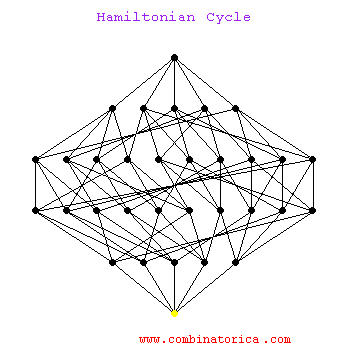## Hamiltonian Cycle / Gray Code Animation

A Hamiltonian Cycle is a cycle that visits each node exactly once. Here we show a Hamiltonian cycle on a 5-dimensional hypercube. Note that it starts by completely traversing the 4-dimensional hypercube on the left before reversing the traversal on the right subcube.

Hamiltonian cycles on hypercubes provide constructions for Gray codes, namely orderings of all subsets of n items such that neighboring subsets differ in exactly one element.

Hamilitonian cycle is an NP-complete problem, so no worst-case efficient algorithm exists to find such a cycle. In practice, we can find Hamiltonian cycles in modest-sized graphs by using backtracking with clever pruning to reduce the search space.